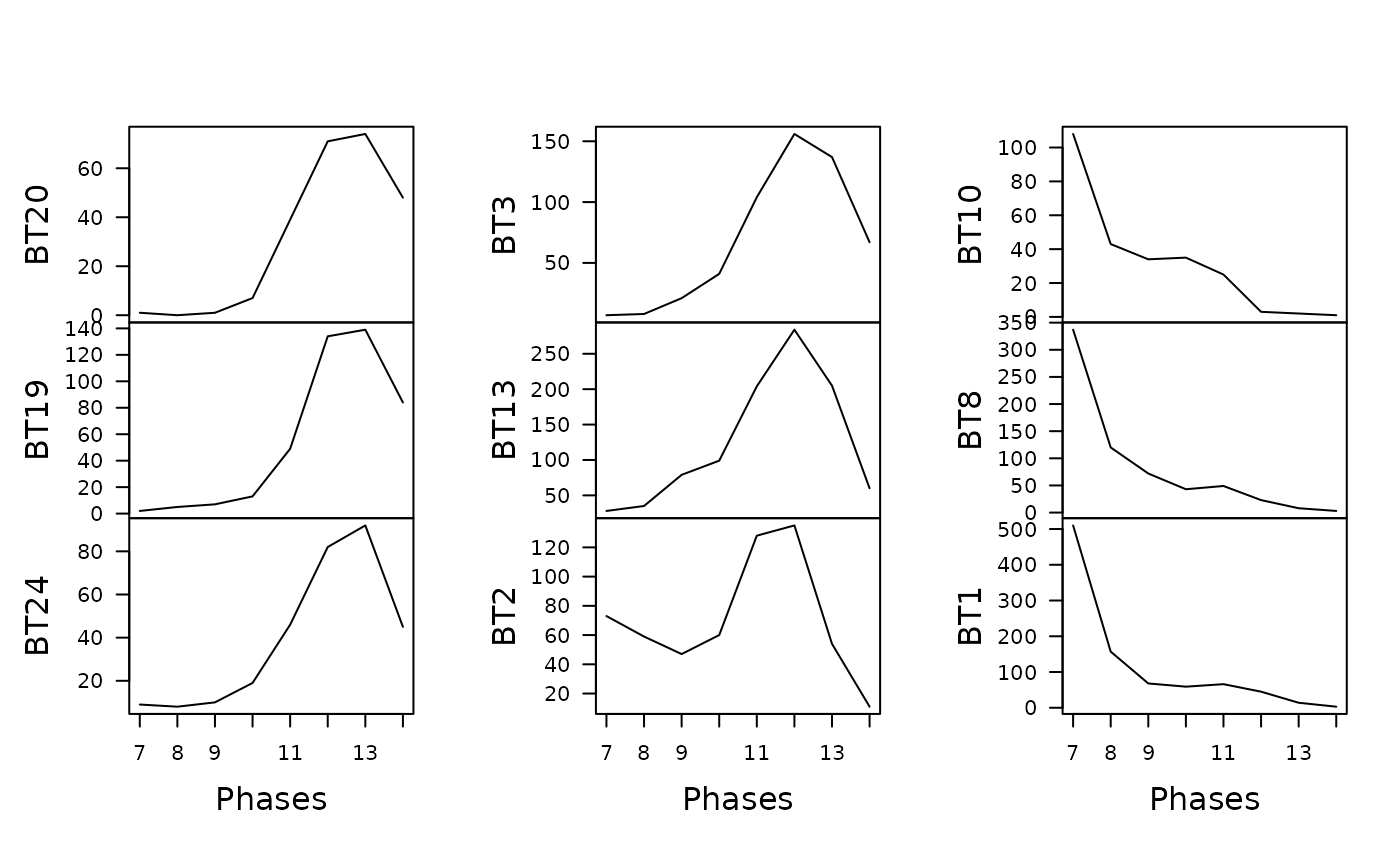Produces an abundance vs time diagram.

## Usage

plot_time(object, dates, ...)

# S4 method for data.frame,numeric
plot_time(object, dates, facet = FALSE)

# S4 method for matrix,numeric
plot_time(object, dates, facet = FALSE)

## Arguments

object

An $$m \times p$$ numeric matrix or a data.frame of count data (absolute frequencies).

dates

A numeric vector of dates.

...

Currently not used.

facet

A logical scalar: should a matrix of panels defined by type/taxon be drawn?

## Value

A ggplot object.

Other plotting methods: plot_aoristic, plot_event, plot_fit, plot_mcd

N. Frerebeau

## Examples

data("merzbach", package = "folio")

## Coerce the merzbach dataset to a count matrix
## Keep only decoration types that have a maximum frequency of at least 50
keep <- apply(X = merzbach, MARGIN = 2, FUN = function(x) max(x) >= 50)
counts <- merzbach[, keep]

## Set dates
## We use the row names as time coordinates (roman numerals)
dates <- as.numeric(utils::as.roman(rownames(counts)))

## Plot abundance vs time
plot_time(counts, dates)plot_time(counts, dates, facet = TRUE)